Application to Computer Vision

## Computer vision¶

The `vision` module of the fastai library contains all the necessary functions to define a Dataset and train a model for computer vision tasks. It contains four different submodules to reach that goal:

Each of the four module links above includes a quick overview and examples of the functionality of that module, as well as complete API documentation. Below, we'll provide a walk-thru of end to end computer vision model training with the most commonly used functionality.

## Minimal training example¶

First, import everything you need from the fastai library.

```from fastai.vision import *
```

First, create a data folder containing a MNIST subset in `data/mnist_sample` using this little helper that will download it for you:

```path = untar_data(URLs.MNIST_SAMPLE)
path
```
`PosixPath('/home/ubuntu/.fastai/data/mnist_sample')`

Since this contains standard `train` and `valid` folders, and each contains one folder per class, you can create a `DataBunch` in a single line:

```data = ImageDataBunch.from_folder(path)
```

You load a pretrained model (from `vision.models`) ready for fine tuning:

```learn = cnn_learner(data, models.resnet18, metrics=accuracy)
```

And now you're ready to train!

```learn.fit(1)
```
Total time: 00:09

epoch train_loss valid_loss accuracy
1 0.140444 0.097685 0.968597

Let's look briefly at each of the `vision` submodules.

## Getting the data¶

The most important piece of `vision.data` for classification is the `ImageDataBunch`. If you've got labels as subfolders, then you can just say:

```data = ImageDataBunch.from_folder(path)
```

It will grab the data in a train and validation sets from subfolders of classes. You can then access that training and validation set by grabbing the corresponding attribute in `data`.

```ds = data.train_ds
```

## Images¶

That brings us to `vision.image`, which defines the `Image` class. Our dataset will return `Image` objects when we index it. Images automatically display in notebooks:

```img,label = ds
img
```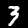You can change the way they're displayed:

```img.show(figsize=(2,2), title='MNIST digit')
```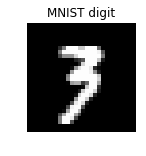And you can transform them in various ways:

```img.rotate(35)
```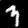## Data augmentation¶

`vision.transform` lets us do data augmentation. Simplest is to choose from a standard set of transforms, where the defaults are designed for photos:

```help(get_transforms)
```
```Help on function get_transforms in module fastai.vision.transform:

get_transforms(do_flip: bool = True, flip_vert: bool = False, max_rotate: float = 10.0, max_zoom: float = 1.1, max_lighting: float = 0.2, max_warp: float = 0.2, p_affine: float = 0.75, p_lighting: float = 0.75, xtra_tfms: Union[Collection[fastai.vision.image.Transform], NoneType] = None) -> Collection[fastai.vision.image.Transform]
Utility func to easily create a list of flip, rotate, `zoom`, warp, lighting transforms.

```

...or create the exact list you want:

```tfms = [rotate(degrees=(-20,20)), symmetric_warp(magnitude=(-0.3,0.3))]
```

You can apply these transforms to your images by using their `apply_tfms` method.

```fig,axes = plt.subplots(1,4,figsize=(8,2))
for ax in axes: ds.apply_tfms(tfms).show(ax=ax)
```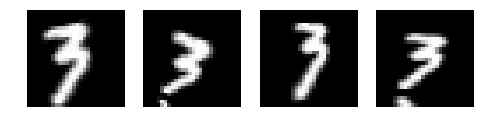You can create a `DataBunch` with your transformed training and validation data loaders in a single step, passing in a tuple of (train_tfms, valid_tfms):

```data = ImageDataBunch.from_folder(path, ds_tfms=(tfms, []))
```

## Training and interpretation¶

Now you're ready to train a model. To create a model, simply pass your `DataBunch` and a model creation function (such as one provided by `vision.models` or `torchvision.models`) to `cnn_learner`, and call `fit`:

```learn = cnn_learner(data, models.resnet18, metrics=accuracy)
learn.fit(1)
```
Total time: 00:08

epoch train_loss valid_loss accuracy
1 0.194779 0.131709 0.950932

Now we can take a look at the most incorrect images, and also the classification matrix.

```interp = ClassificationInterpretation.from_learner(learn)
```
```interp.plot_top_losses(9, figsize=(6,6))
```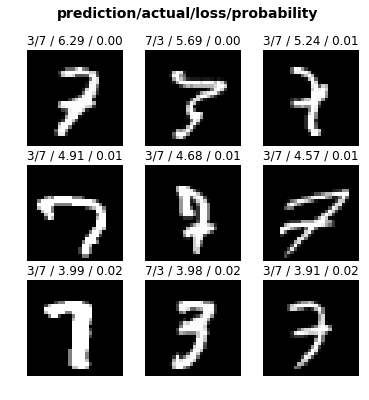```interp.plot_confusion_matrix()
```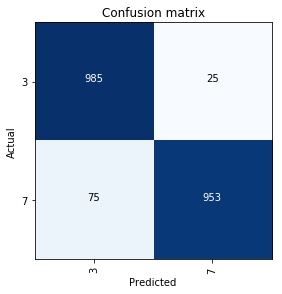To simply predict the result of a new image (of type `Image`, so opened with `open_image` for instance), just use `learn.predict`. It returns the class, its index and the probabilities of each class.

```img = learn.data.train_ds
learn.predict(img)
```
`(Category 3, tensor(0), tensor([0.5551, 0.4449]))`